Next: Null Parameters and Changing Up: Derivation of Similarity Function Previous: Derivation of Similarity Function

## Intensity Parameters: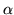This posterior form above can be integrated in the case where all the parameters,, are associated with a column in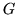that is linearly independent and has a norm greater than one. However, this is not commonly the case, and the set of parameters must be split into subsets. These subsets (see figure 2) are: interesting (int), for shapes inside the valid field of view (i.e. contained in the observed image); null (null), for shapes totally outside the field of view; uninteresting (un), for voxels inside the field of view but not within a modelled shape (i.e. in `areas of no interest'); and partial volume (pv), for shapes that overlap the field of view by less than one voxel. That is: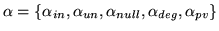Figure 2: Example of shape models superimposed on an image showing (in one 2D coronal slice); Field of View (FOV), Shapes of Interest (INT), Areas of No Interest (ANI), Null Shapes (NULL), and Partial Volume Shapes (PV). Note that all parts of the observed image not covered by a shape model is part of the Area of No Interest.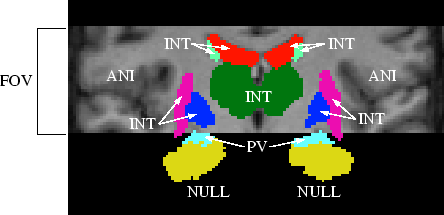The dimensionality of the subsets will be denoted as: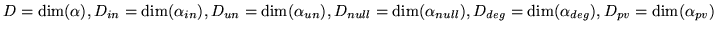such that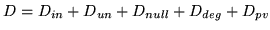. Note that the composition and dimension of these subsets depends heavily on the spatial transformation,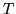. Hence, like, they implicitly encode dependence on, but asis not being marginalised, this dependence will be left implicit. It is important to remember, however, that these are not constants.

Also, many of these subsets may be empty (zero dimensional) for certain spatial transformations,, or always empty in certain applications (e.g. where there are no areas of no interest). The way that these subsets are defined and how they are treated in the integrations will be the topic of the next sections.

SubsectionsNext: Null Parameters and Changing Up: Derivation of Similarity Function Previous: Derivation of Similarity Function